# Crustal thickness recovery using an isostatic model and GOCE data

## Abstract

One of the GOCE satellite mission goals is to study the Earth’s interior structure including its crustal thickness. A gravimetric-isostatic Moho model, based on the Vening Meinesz-Moritz (VMM) theory and GOCE gradiomet ric data, is determined beneath Iran’s continental shelf and surrounding seas. The terrestrial gravimetric data of Iran are also used in a nonlinear inversion for a recovering-Moho model applying the VMM model. The newly-computed Moho models are compared with the Moho data taken from CRUST2.0. The root-mean-square (RMS) of differences between the CRUST2.0 Moho model and the recovered model from GOCE and that from the terrestrial gravimetric data are 3.8 km and 4.6 km, respectively.

## 1. Introduction

The Mohorovičić discontinuity usually called the Moho, is the boundary between the Earth’s crust and mantle. This boundary can be determined by isostatic/gravimetric and seismic methods. Several isostatic hypotheses and seismic models exist for estimating the crustal thickness/Moho. The isostatic models are well-known from the literature (see e.g. Heiskanen and Moritz, 1967, p. 133; Moritz, 1990, chapter 8; Sjöberg, 2009; Bagherbandi, 2011). A comparison between different classical Moho models, and a Moho model determined from seismic data, was presented in Bagherbandi (2011). The advantage of using an isostatic/gravimetric model to determine the crustal thickness is the uniform coverage and relatively-detailed resolution of the currently-available global geopotential models and satellite data, especially over large areas of the world where seismic data are not available or their spatial coverage is not sufficient.

According to our knowledge, there exist few studies, based on satellite data, to determine the crustal thickness. In Shin et al. (2007), a recovery of the Moho depth using a geopotential model obtained from a Gravity Recovery and Climate Experiments (GRACE) satellite mission (Tapley et al., 2005) was presented to determine the Moho depth beneath Tibet. Sampietro (2009) considered the local inversion of Satellite Gravity Gradiometry (SGG) data by simulating a Moho surface and generating the SGG data based on that. Bagherbandi (2011) studied a Moho model obtained from the Vening Meinesz-Moritz (VMM) model (Sjöberg, 2009) and simulated SGG data by EGM08 (Pavlis et al., 2008) in the presence of a white noise of 10 mE (1 mE = 0.0001 mGal/km).

The Gravity field and steady-state Ocean Circulation Explorer (GOCE) (see ESA, 1999) could deliver Earth’s gravity fields to degree and order 250 in its spherical harmonic expression. Here, our purpose is to use real GOCE data directly for determining a regional Moho model, and not their products as the geopotential models. The downward continuation of the GOCE gradiometric data and the regional recovery of the Moho depth is performed simultaneously using the VMM model (Sjöberg, 2009) through a nonlinear integral inversion procedure.

## 2. Development of the Vening Meinesz-Moritz Method for GOCE Data

The principle of the VMM theory is the same as that of Vening Meinesz which assumes that the Bouguer gravity anomaly, Δg B , at any point P, is compensated by an attraction of A C so that the isostatic gravity anomaly, Δg I , vanishes. Mathematically this idea is described by (Sjöberg, 2009):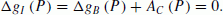(1)

Sjöberg (2009) presented some solutions to determine A C by dividing it into two parts: a mean depth and fluctuations of the Moho surface around it; see Sjöberg (2009, equation 53b) for the mathematical derivations.

In a similar manner, Bagherbandi (2011) modified Eq. (1) to recover the second-order radial derivative of the compensation potential instead of the compensation attraction: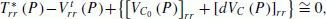(2)

where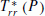is the second-order radial derivative of the disturbing potential T * (P) at a point P and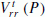stands for its topographic effect (see e.g. Wild and Heck, 2004a, b; Eshagh and Sjöberg, 2008; Bagherbandi, 2011, chap. 4).and [dV C (P)] rr are the second-order radial derivatives of the potentials of the shell with thickness of the mean depth of Moho, and its variable part, respectively. Equation (2) can easily be developed to other types of gradients depending on their describing frame (cf. Bagherbandi, 2011, chap. 5). However, since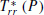has the strongest signal and the simplest mathematical form with respect to the other gradients, it is used in this study.

The Moho depth fluctuations being recovered are nonlinear inside [dV C (P)] rr . We have to assume that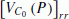is already known from external sources. This term compensates the majority of the signal of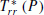and the residuals of this compensation are those related to the variation of Moho around its mean value. Equation (2) can be rewritten so as to have the unknown parameters on one side (Bagherbandi, 2011):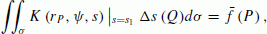(3a)

where σ is the unit sphere, the surface integration element, Δs the corrections to the approximate value s1 connecting to the Moho depth T by s = (1 − T / R) and R is the radius of a sphere equal to the semi-major axis of the reference ellipsoid, ψ stands for the geocentric angle between the computation point P and the integration (dummy) point Q, r P is the geocentric distance of P, and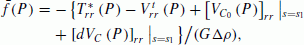(3b)

where G = 6.674 × 10−11 m3 kg−1 s−2 is Newton’s gravitational constant, Δ ρ denotes the crust-mantle density constant, and the integral kernel of integration can be expressed as: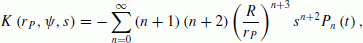(3c)

where P n (t) is the Legendre polynomial of degree n for the argument t = cos ψ. The closed analytical form of the kernel in Eq. (3c) was presented by Bagherbandi (2011).

As observed, the unknown parameter s is inside the kernel function of the integral equations. Therefore, Eq. (3a) is categorized in the nonlinear integral equations requiring the approximate values of s. In fact, the Moho undulations will be added to these approximate values by iterating the inversion. This is the reason for the appearance of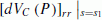in the right-hand side of Eq. (3a), if the integral equation was linear, this term would not appear.

Equation (3a) can be written in the following matrix form: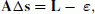(4)

where A stands for the coefficient matrix obtained by the discrete form of the integral, Δs is the vector of the unknown containing the increments of the Moho depths. L is the vector obtained from Eq. (3b) and ε stands for errors.

Equation (4) is not an ordinary system because it was derived from discretising integral formulas. It is well-known that such a system is ill-conditioned and its solution is highly dependent on the error of the data. Even small errors in input data can significantly change the results. In order to control the stability of the inversion process, the system should be regularised and a smooth solution should be sought. Tikhonov (1963) was one of the first to suggest the idea of adding a small positive number to the diagonal elements of the coefficients matrix of the normal equations for the purpose of stabilisation. The consequence of this action is to have a biased solution, which is the penalty of the stabilisation. The regularised solution of Eq. (4) is: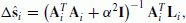(5a)

where α2 is the so-called regularization parameter and I denotes the identity matrix. The subscript i stands for the iteration and updating the vectors and matrices. Now, if we assume thatis the solution of Eq. (4), then the updated value of s2 is obtained by s2 = s1 + Δŝ1 and the computations are repeated using Eqs. (3a) and (3b) with the new value of s2. This procedure is repeated until the difference between the last two solutions is smaller than a level of convergence. The bias of regularized solution can be estimated by (Bouman, 1998, p. 27; Xu, 1998; Eshagh, 2009):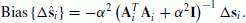(5b)

One important issue in the Tikhonov regularization is the proper selection of the regularization parameter α2. There are methods for computing it, such as the L-curve, generalized cross-validation, and the quasi-optimality methods (Hansen, 1998, 2008).

Once the solution ŝ is found, the computation of the Moho depth T is straightforward from: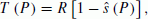(5c)

Due to the nonlinear nature of the problem the solution should be iterated and the Moho depths should be updated iteratively. For details about the convergence of the solution, the effect of the spatial truncation error, and other practical issues concerning the nonlinear inversion of Eq. (3a), we refer readers to Bagherbandi (2011).

## 3. Numerical Realization

Here, we select Iran, restricted between latitudes 19° and 46°N and longitudes 19° and 46°E as our study area. Values of 650 and 430 kg m−3 were taken for the crust-mantle density contrast in land and sea areas, according to Sjöberg and Bagherbandi (2011). Mean Moho depths of 37 km and 23 km were obtained from the spatial averaging of the 2° × 2° CRUST2.0 (Bassin et al., 2000) Moho depths for the corresponding areas, respectively (see, for example, the discussion concerning the seismic method and its accuracy in Nakamura and Umedu, 2009). Our goal is to determine 2° × 2° Moho models with the same resolution as that of CRUST2.0, from 1° × 1° GOCE and terrestrial gravimetric data. Two gravimetric models for Moho beneath this area are computed. One based on Sjöberg’s (2009, equation 53b) direct integral approach using terrestrial data, and the other one based on solving the nonlinear inversion method presented in the previous section. Finally, both models are compared to each other and to that with CRUST2.0 data. Here, a larger area by 5° is considered for the recovery which is required to reduce the effect of the truncation error of the integral formula in the inversion method (Eq. (4)) but the results of the central part are selected. Due to the nonlinear nature of the problem the approximate value outside the central area are not updated for the reduction of this truncation error. According to Bagherbandi (2011), three iterations are required to reach the acceptable convergence level, i.e. 50 m, and removing the bias of regularization, Eq. (5b), has an essential role for solving the problem.

Here, only the second-order radial derivative of the geopotential observed by GOCE in December 2009 (GOCE.EGG.TRF_2; ESA, 2008) is used for our recovery purpose. This product (EGG_TRF_2) contains the L2 gravity gradients in the LNOF (Local North Oriented Frame). In order to change to the derivative of the disturbing potential, the normal gravity field GRS80 was used to generate the normal gradients at the same positions of the GOCE data and are then subtracted from them. The results vary from 0.97E to −1.08E with the mean of 0.01E and the standard deviation is 0.39E. The data are gridded 1° × 1° by interpolation and continued downward to a level of 250 km above sea level. The EGM08 coefficients were model (Pavlis et al., 2008) was used for estimating the downward continuation effect of the gradients to that level. Eshagh (2011) has shown that the results of the inversion of gridded gradients are more successful than those of an on-orbit inversion due to the better conditionality of the coefficient matrix being inverted. The map of the gradients at this level is presented in Fig. 1(a) showing large, and positive, values for the gradients over mountainous areas, and large, but negative, values above seas. The approximate Moho depths, based on the VMM theory (Sjöberg, 2009, equation 50), which are needed for initiating both Sjöberg’s direct solution with terrestrial data, and the nonlinear inversion method, are presented in Fig. 1(b). The maximum, mean, minimum and standard deviation of the depths are 53.7, 36.3, 18.7 and 4.9 in unit of km, respectively. There are similarities between the maps of gradients and approximate Moho depths as both are influenced by the topographic features in Iran. The topographic effect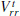should be removed from T rr so that the result reflects solely the changes of the Earth’s interior including Moho’s variations. The topographic model DMT2006 to degree/order 180 (Pavlis et al., 2007) was used to compute. The computed values ofvary from 3.46E to −0.75E with a standard deviation of 0.91E in the territory.

The terrestrial gravimetric data provided by the National Cartographic Centre (NCC) of Iran, and Abdollahzadeh and Najafi (2008), detected gross-errors and cleaned them for geoid determination purposes. Here, the model recovered using the terrestrial data and the direct method is denoted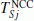and TGOCE, respectively. Figures 2(a), 2(b) and 2(c) show the maps of TGOCE,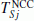and the Moho model of CRUST2.0, TRUST2.0. According to Figs. 2(a) and 2(b), a smoother model of Moho is recovered from the GOCE data than that obtained from the terrestrial gravimetric data. However, Fig. 2(c) shows also a smooth model of Moho for the area. The high altitude of the satellite, and regularization as a smoothing method, could be possible reasons for resulting in a smooth surface which is closer to the smooth model of TCRUST2.0.

Statistics of the Moho models and their differences are summarized in Table 1. The mean of TGOCE is larger than that of, which means that TGOCE is deeper because we think that the local topographic effects (some of which disturb gravity signals) still remind on the terrestrial data and this can affect the Moho results significantly. Therefore, the SGG data are smoother and better than the terrestrial data, because of the latitude of the GOCE satellite. The table shows that TGOCE derived from the GOCE data is closer to the CRUST2.0 Moho depths TCRUST2.0. However, it should be mentioned that the gravimetric and seismic Moho depths are not necessarily the same. The unrealistic assumptions of a constant density contrast between the crust and mantle, on one hand, neglecting some geophysical phenomena, such as mantle convection/thermal compensation can be possible reasons for their separation. Also, Martinec (1994) has pointed out that other compensation mechanisms exist in addition to the isostatic one, for unformulated phenomena (see also Yoshida, 2004).Table 1 Statistics of Moho depths and their differences, Sjöberg’s direct solution (), the Moho recovered from GOCE data (TGOCE) and the seismic Moho (TCRUST2.0). Unit: 1 km.

## 4. Conclusions

We have used the Vening Meinesz-Moritz theory and GOCE data, to compute and analyze the crust thickness beneath Iran’s continental shelf and surrounding areas. Here, the Vening Meinesz-Moritz theory has been further developed so that the satellite gravity gradiometry (SGG) data can be used for recovering the Moho depth through a nonlinear integral inversion procedure. The kernels of its forward and inverse problems showed that the inversion should be performed in an area larger by 5° than the desired one to reduce the effect of the spatial truncation error of the integral formula. The results were compared with the CRUST2.0 data. Our numerical study showed that the effect of the truncation error on the recovered Moho depths can attain 6 km in Iran, and this is very significant. The iterative Tikhonov regularization in combination with either the generalized cross-validation, or quasi-optimal, criterion of estimating the regularization parameter seems to be suitable and the solution is semi-convergent up to the third iteration. The Moho depth recovered from GOCE data was the same as that obtained from the terrestrial data with a root-mean-square error of 4.56 km. The results revealed the significant correlation of the Moho geometry with the seismic model, CRUST2.0. The root-mean-square error of the recovered Moho from GOCE with CRUST2.0 is 3.78 km.

## References

1. Abdollahzadeh, M. and M. Najafi Almdari, Detection of Gross-Errors into Gravity Data of IRAN by Kriging Approach, SGM 2009 Abstract Vol Book V, presented in 6th Swiss Geoscience Meeting (2008), Lugano, Switzerland, pp. 297–298, 2008.

2. Bagherbandi, M., An isostatic Earth crustal model and its application, Ph.D. Dissertation, Royal Institute of Technology (KTH), Stockholm, Sweden, 207 pp., 2011.

3. Bassin, C., G. Laske, and T. G. Mastersm, The current limits of resolution for surface wave tomography in North America, EOS Trans. AGU, 81, F897, 2000.

4. Bouman, J., Quality of regularization methods, DECS Report no. 98.2, Delft University Press, 1998.

5. ESA, Gravity field and steady-state ocean circulation mission, ESA SP-1233(1), Report for Mission Selection of the Four Candidate Earth Explorer Missions, pp. 217, ESA Publications Division, July 1999, 1999.

6. ESA, GOCE Level 2 Product Data Handbook, Doc. No.: GO-MA-HPF-GS-0110. Issue: 4 Date: 09/06/2008, prepared by: The European GOCE Gravity Consortium EGG-C, 2008.

7. Eshagh, M., On satellite gravity gradiometry, Doctoral dissertation in Geodesy, Royal Institute of Technology (KTH), Stockholm, Sweden, 2009.

8. Eshagh, M., The effect of spatial truncation error on integral inversion of satellite gravity gradiometry data, Adv. Space Res., 47, 1238–1247, 2011.

9. Eshagh, M. and L. E. Sjöberg, Impact of topographic and atmospheric masses over Iran on validation and inversion of GOCE gradiometric data, Earth Space Phys., 34, 15–30, 2008.

10. Hansen, P. C., Rank-deficient and discrete ill-posed problems: numerical aspects of linear inversion, SIAM, Philadelphia, 1998.

11. Hansen, P. C., Regularization Tools version 4.0 for Matlab 7.3, Numer. Algorithms, 46, 189–194, 2008.

12. Heiskanen, W. and H. Moritz, Physical Geodesy, W.H. Freeman and company, San Francisco and London, 1967.

13. Martinec, Z., The minimum depth of compensation of topographic masses, Geophys. J. Int., 117, 545–554, 1994.

14. Moritz, H., The Figure of the Earth, 277 pp., H Wichmann, Karlsruhe, 1990.

15. Nakamura, M. and N. Umedu, Crustal thickness beneath the Ryukyu arc from travel-time inversion, Earth Planets Space, 61, 1191–1195, 2009.

16. Pavlis, N. K., J. K. Factor, and S. A. Holmes, R. Forsberg, Proceedings of the 1st International Symposium of the International Gravity Field Service (IGFS), Harita Dergisi, Special Issue No. 18, General Command of Mapping, Ankara, Turkey, 2007

17. Pavlis, N., S. A. Holmes, S. C. Kenyon, and J. K. Factor, An Earth Gravitational model to degree 2160: EGM08, presented at the 2008 General Assembly of the European Geosciences Union, (Vienna, Austria), April 13–18, 2008.

18. Sampietro, D., An inverse gravimetric problem with GOCE data, Ph.D. thesis, Politecnico di Milano Polo regionale di Como. 2009.

19. Shin, Y. H., H. Xu, C. Braitenberg, J. Fang, and Y. Wang, Moho undulations beneath Tibet from GRACE-integrated gravity data, Geophys. J. Int., 170, 971–985, 2007.

20. Sjöberg, L. E., Solving Vening Meinesz-Moritz inverse problem in isostasy, Geophys. J. Int., 179 (3), 1527–1536, 2009.

21. Sjöberg, L. E. and M. Bagherbandi, A method of estimating the Moho density contrast with a tentative application by EGM08 and CRUST2.0, Acta Geophys., 59 (3), 502–525, 2011.

22. Tapley, B., J. Ries, S. Bettadpur, D. Chambers, M. Cheng, F. Condi, B. Gunter, Z. Kang, P. Nagel, R. Pastor, T. Pekker, S. Poole, and F. Wang, GGM02-An improved Earth gravity field model from GRACE, J Geod., 79, 467–478, 2005.

23. Tikhonov, A. N., Solution of incorrectly formulated problems and regularization method, Soviet Math. Dokl., 4, 1035–1038, English translation of Dokl. Akad. Nauk. SSSR, 151, 501–504, 1963.

24. ai]Wild, F. and B. Heck, A comparison of different isostatic models applied to satellite gravity gradiometry, Gravity, Geoid and Space Missions GGSM 2004 IAG International Symposium Porto, Portugal August 30–September 3, 2004a.

25. Wild, F. and B. Heck, Effects of topographic and isostatic masses in satellite gravity gradiometry, in Proc. Second International GOCE User Workshop GOCE. The Geoid and Oceanography, ESA-ESRIN, Frascati/Italy, March 8–10, 2004 (ESA SP–569, June 2004), CD-ROM, 2004b.

26. Xu, P., Truncated SVD methods for discrete linear ill-posed problems, Geophys. J. Int., 135, 505–514, 1998.

27. Yoshida, M., Influence of two major phase transitions on mantle convection with moving and subducting plates, Earth Planets Space, 56, 1019–1033, 2004.

## Acknowledgements

The authors would like to thank Professor Lars E. Sjöberg for his guidance and help. The unknown reviewers are cordially thanked for their constructive comments on the manuscript. Mohammad Bagherbandi and Mehdi Eshagh were supported by Projects no. 76/10:1 and 98/09:1 of the Swedish National Space Board (SNSB), respectively. Mr. Mohsen Romeshkani and Mr. Makan Abdolahzadeh are thanked for preparing the SGG data and terrestrial gravity data for this work.

Authors

## Rights and permissions

Reprints and Permissions

Bagherbandi, M., Eshagh, M. Crustal thickness recovery using an isostatic model and GOCE data. Earth Planet Sp 64, 1053–1057 (2012). https://doi.org/10.5047/eps.2012.04.009

• Revised:

• Accepted:

• Published:

• Issue Date:

### Key words

• Isostasy
• Tikhonov regularization
• Moho
• nonlinear ill-posed problem